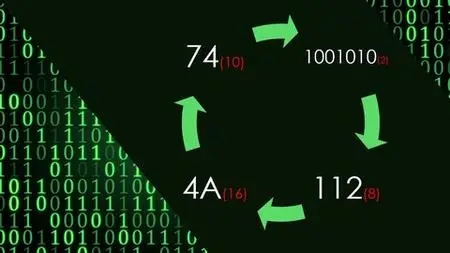Numbers conversion (Dec/Bin/Oct/Hex) and binary arithmeticsNumbers conversion (Dec/Bin/Oct/Hex) and binary arithmetics
.MP4 | Video: 1280×720, 30 fps(r) | Audio: AAC, 44100 Hz, 2ch | 661 MB
Duration: 2 hours | Genre: eLearning Video | Language: English

Convert from/to decimal, binary, octal, hexadecimal, IEEE 754, BCD, ASCII and gray, and do binary arithmetics (+ – * /) .

Number systems concept

Binary arithmetic operations (+ – * /)

Convert from/to decimal, binary, octal, hexadecimal, IEEE 754, BCD, ASCII and gray, and do binary arithmetics (+ – * /) .
What you’ll learn
Number systems concept
Decimal, binary, octal and hexadecimal conversions
Binary arithmetic operations (+ – * /)
IEEE 754, BCD, ASCII and gray conversions
Requirements
Just a sheet and a pen to practise
Description
This is a course about numbers conversion and binary arithmetics, it contains 44 lectures for a total of 2 hours
Why would you take this course :
– Simple explications
– A lot of examples/practise